# Trimesh#

## Triangle Meshes#

Along with points, timeseries, trajectories, and structured grids, Datashader can rasterize large triangular meshes, such as those often used to simulate data on an irregular grid:Any polygon can be represented as a set of triangles, and any shape can be approximated by a polygon, so the triangular-mesh support has many potential uses.

In each case, the triangular mesh represents (part of) a surface, not a volume, and so the result fits directly into a 2D plane rather than requiring 3D rendering. This process of rasterizing a triangular mesh means generating values along specified regularly spaced intervals in the plane. These examples from the Direct3D docs show how this process works, for a variety of edge cases:This diagram uses “pixels” and colors (grayscale), but for datashader the generated raster is more precisely interpreted as a 2D array with bins, not pixels, because the values involved are numeric rather than colors. (With datashader, colors are assigned only in the later “shading” stage, not during rasterization itself.) As shown in the diagram, a pixel (bin) is treated as belonging to a given triangle if its center falls either inside that triangle or along its top or left edge.

The specific algorithm used to do so is based on the approach of Pineda (1998), which has the following features:

• Classification of pixels relies on triangle convexity

• Embarrassingly parallel linear calculations

• Inner loop can be calculated incrementally, i.e. with very “cheap” computations

and a few assumptions:

• Triangles should be non overlapping (to ensure repeatable results for different numbers of cores)

• Triangles should be specified consistently either in clockwise or in counterclockwise order of vertices (winding).

Trimesh rasterization is not yet GPU-accelerated, but it’s fast because of Numba compiling Python into SIMD machine code instructions.

## Tiny example#

To start with, let’s generate a tiny set of 10 vertices at random locations:

```import numpy as np, datashader as ds, pandas as pd
from scipy.spatial import Delaunay

n = 10
np.random.seed(2)

x = np.random.uniform(size=n)
y = np.random.uniform(size=n)
z = np.random.uniform(0,1.0,x.shape)

pts = np.stack((x,y,z)).T
verts = pd.DataFrame(np.stack((x,y,z)).T, columns=['x', 'y' , 'z'])
```

Here we have a set of random x,y locations and associated z values. We can see the numeric values with “head” and plot them (with color for z) using datashader’s usual points plotting:

```cvs = ds.Canvas(plot_height=400,plot_width=400)

```

x y z
0 0.435995 0.621134 0.505246
1 0.025926 0.529142 0.065287
2 0.549662 0.134580 0.428122
3 0.435322 0.513578 0.096531
4 0.420368 0.184440 0.127160
5 0.330335 0.785335 0.596745
6 0.204649 0.853975 0.226012
7 0.619271 0.494237 0.106946
8 0.299655 0.846561 0.220306
9 0.266827 0.079645 0.349826
Points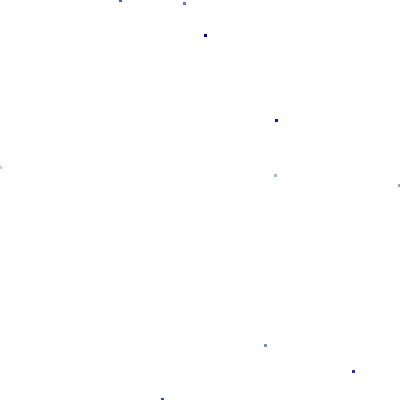To make a trimesh, we need to connect these points together into a non-overlapping set of triangles. One well-established way of doing so is Delaunay triangulation:

```def triangulate(vertices, x="x", y="y"):
"""
Generate a triangular mesh for the given x,y,z vertices, using Delaunay triangulation.
For large n, typically results in about double the number of triangles as vertices.
"""
triang = Delaunay(vertices[[x,y]].values)
print('Given', len(vertices), "vertices, created", len(triang.simplices), 'triangles.')
return pd.DataFrame(triang.simplices, columns=['v0', 'v1', 'v2'])
```
```%time tris = triangulate(verts)
```
```Given 10 vertices, created 12 triangles.
CPU times: user 1.76 ms, sys: 109 µs, total: 1.86 ms
Wall time: 5.04 ms
```

The result of triangulation is a set of triangles, each composed of three indexes into the vertices array. The triangle data can then be visualized by datashader’s `trimesh()` method:

```tf.Images(tris.head(15), tf.shade(cvs.trimesh(verts, tris)))
```

v0 v1 v2
0 2 4 9
1 9 4 1
2 4 3 1
3 3 4 7
4 4 2 7
5 8 5 7
6 5 8 6
7 5 6 1
8 3 0 1
9 0 5 1
10 0 3 7
11 5 0 7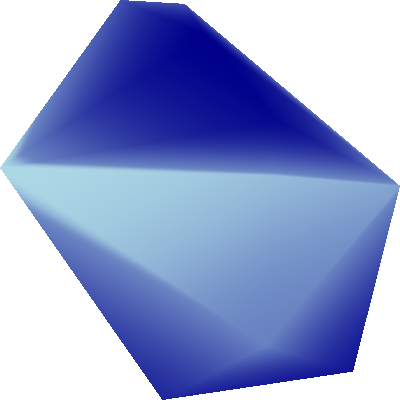By default, datashader will rasterize your trimesh using z values linearly interpolated between the z values that are specified at the vertices. The shading will then show these z values as colors, as above. You can enable or disable interpolation as you wish:

```from colorcet import rainbow as c
tf.Images(tf.shade(cvs.trimesh(verts, tris, interpolate='nearest'), cmap=c, name='10 Vertices'),
tf.shade(cvs.trimesh(verts, tris, interpolate='linear'),  cmap=c, name='10 Vertices Interpolated'))
```
 10 Vertices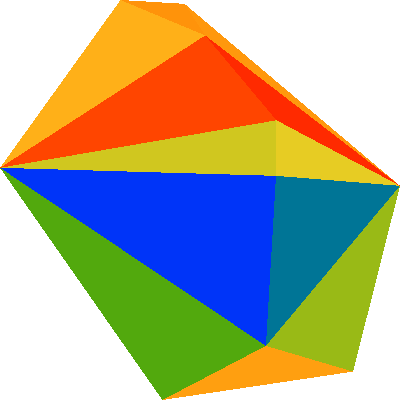10 Vertices Interpolated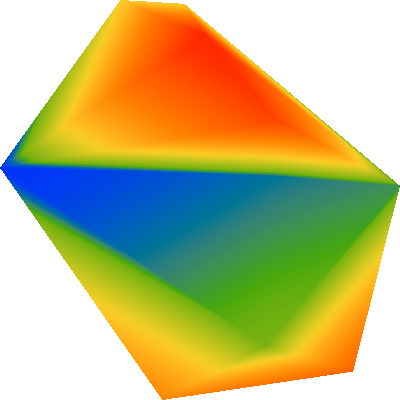## More complex example#

The small example above should demonstrate how triangle-mesh rasterization works, but in practice datashader is intended for much larger datasets. Let’s consider a sine-based function `f` whose frequency varies with radius:

```rad = 0.05,1.0

def f(x,y):
rsq = x**2+y**2
```

We can easily visualize this function by sampling it on a raster with a regular grid:

```n = 400

ls  = np.linspace(-1.0, 1.0, n)
x,y = np.meshgrid(ls, ls)
img = f(x,y)

raster
```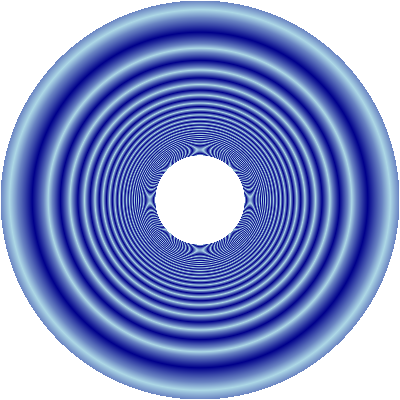However, you can see pronounced aliasing towards the center of this function, as the frequency starts to exceed the sampling density of the raster. Instead of sampling at regularly spaced locations like this, let’s try evaluating the function at random locations whose density varies towards the center:

```def polar_dropoff(n, r_start=0.0, r_end=1.0):
ls = np.linspace(0, 1.0, n)
ex = np.exp(2-5*ls)/np.exp(2)
theta  = np.random.uniform(0.0,1.0, n)*np.pi*2.0
x = radius * np.cos( theta )
y = radius * np.sin( theta )
return x,y

z = f(x,y)

verts = pd.DataFrame(np.stack((x,y,z)).T, columns=['x', 'y' , 'z'])
```

We can now plot the x,y points and optionally color them with the z value (the value of the function f(x,y)):

```cvs = ds.Canvas(plot_height=400,plot_width=400)

```
 Points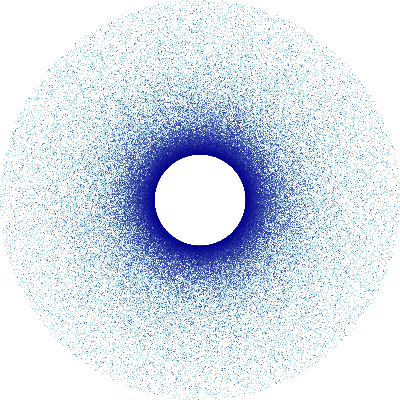PointsZ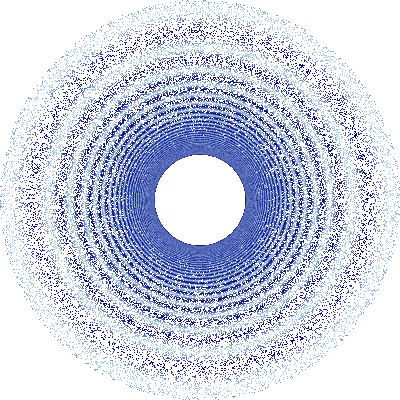The points are clearly covering the area of the function that needs dense sampling, and the shape of the function can (roughly) be made out when the points are colored in the plot. But let’s go ahead and triangulate so that we can interpolate between the sampled values for display:

```%time tris = triangulate(verts)
```
```Given 160000 vertices, created 319914 triangles.
CPU times: user 983 ms, sys: 44 ms, total: 1.03 s
Wall time: 1.03 s
```

And let’s pre-compute the combined mesh data structure for these vertices and triangles, which for very large meshes (much larger than this one!) would save plotting time later:

```%time mesh = du.mesh(verts,tris)
```
```CPU times: user 19 ms, sys: 3.95 ms, total: 22.9 ms
Wall time: 22.7 ms
```

This mesh can be used for all future plots as long as we don’t change the number or ordering of vertices or triangles, which saves time for much larger grids.

We can now plot the trimesh to get an approximation of the function with noisy sampling locally to disrupt the interference patterns observed in the regular-grid version above and preserve fidelity where it is needed. (Usually one wouldn’t do this just for the purposes of plotting a function, since the eventual display on a screen is a raster image no matter what, but having a variable grid is crucial if running a simulation where fine detail is needed only in certain regions.)

```tf.shade(cvs.trimesh(verts, tris, mesh=mesh))
```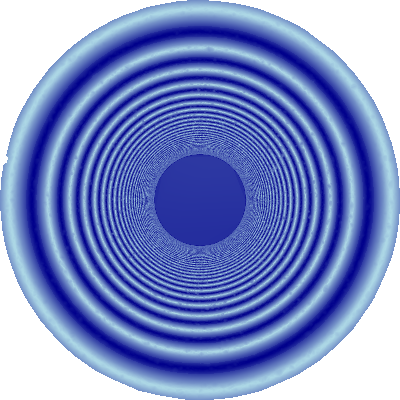The fine detail in the heavily sampled regions is visible when zooming in closer (without resampling the function):

```tf.Images(*([tf.shade(ds.Canvas(x_range=r, y_range=r).trimesh(verts, tris, mesh=mesh))
for r in [(0.1,0.8), (0.14,0.4), (0.15,0.2)]]))
```